# ISEE Upper Level Quantitative : How to find the area of a rectangle

## Example Questions

2 Next →

### Example Question #281 : Plane Geometry

The perimeter of a rectangle is 210 inches. The width of the rectangle is 40% of its length. What is the area of the rectangle?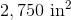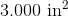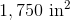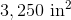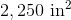Explanation:

If the width of the rectangle is 40% of the length, then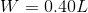.

The perimeter of the rectangle is: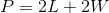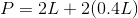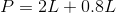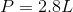The perimeter is 210 inches, so we can solve for the length: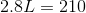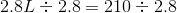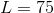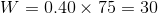The length and width of the rectangle are 75 and 30 inches; the area is their product, or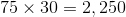square inches.

### Example Question #282 : Plane Geometry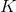is a positive integer.

Rectangle A has length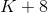and width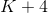; Rectangle B has length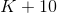and length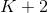. Which is the greater quantity?

(A) The area of Rectangle A

(B) The area of Rectangle B

It is impossible to determine which is greater from the information given

(A) is greater

(A) and (B) are equal

(B) is greater

(A) is greater

Explanation:

This might be easier to solve if you set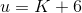.

Then the dimensions of Rectangle A are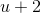and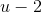. The area of Rectangle A is their product: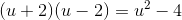The dimensions of Rectangle B are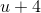and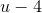.  The area of Rectangle B is their product: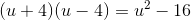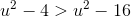regardless of the value of(or, subsequently,), so Rectangle A has the greater area.

### Example Question #13 : How To Find The Area Of A Rectangle

In Rectangle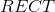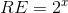and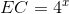. Give the area of this rectangle in terms of.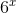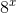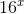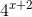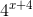Explanation:

The area of a rectangle is the product of its length and its width, which here are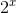and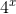. Mulitply: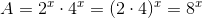2 Next →

### All ISEE Upper Level Quantitative Resources